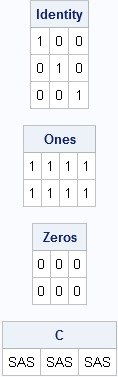In linear algebra, the I symbol is used to denote an n x n identity matrix. The symbol J (or sometimes 1) is used to denote an n x p matrix of ones.

When the SAS/IML language was implemented, the I function was defined to generate the identity matrix. The J function was defined to produce a J matrix by default, but the function accepts an optional third parameter that sets the value of the matrix that is created. You can even use the J fnction to create a character matrix, as follows:

```proc iml; Identity = I(3); Ones = J(2, 4); Zeros = J(2, 3, 0); C = J(1, 3, "SAS"); print Identity, Ones, Zeros, C;```Incidentally, not all matrix languages made the same choice regarding what to name the functions that return special matrices. The MATLAB language has special functions named 'eye', 'ones', and 'zeros.' In R, you can use the 'diag' function to create an identity matrix and the 'matrix' function to create a matrix of constant values.

ShareDistinguished Researcher in Computational Statistics

Rick Wicklin, PhD, is a distinguished researcher in computational statistics at SAS and is a principal developer of SAS/IML software. His areas of expertise include computational statistics, simulation, statistical graphics, and modern methods in statistical data analysis. Rick is author of the books Statistical Programming with SAS/IML Software and Simulating Data with SAS.

•••